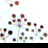# calculating distance matrix for a group of points

3064
4
07-15-2014 12:38 AMNew Contributor II

Hello,

I have a layer with points. Now I´m trying to calculate the distance from each point to all other points with the network analyst. The points are not part of the street network I created. But I want to calculate the distance with by this street network.

The problem is

1. how can i join the points with the network ( how to find the nearest street network node to each point)?

2.how can i calculate the shortest distance form each point to all the other points ?

4 RepliesbyMVP Esteemed Contributor

In many of the analyses in NA, the point file will be either your origins or destinations.  How far are the points from the network?  Did you set up a search tolerance when creating the network?New Contributor II

Do you mean to try to make OD cost matrix?

The points distances from the network are 20-250 m.byMVP Esteemed Contributor

If the points are within the search tolerance that you specified, they should be found.  There is no harm in tryingbyEsri Regular Contributor

OD Cost Matrix is the way to find the network distances between sets of points. And when you do Load Locations or use the GP tool Add Locations, it automatically finds the closest edge the points will snap to. If you just want to know where they will snap to on the network then you can use the GP tool Calculate Locations for that.

Jay Sandhu